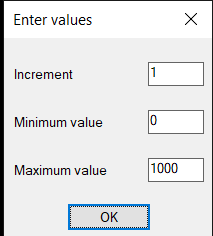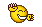# Numeric Text Editing

## Recommended PostsI found this excellent lisp program by Lee Mac and just wondering if there's anyone out there who can help modify the program to increment only the numbers in a certain range instead of all the numbers in a selected text or mtext.  It's like adding a prompt for starting and ending numbers and increment only those within the given range.  Any help would be highly appreciated.

```;; Text Increment  -  Lee Mac
;; Increments numerical data found in a selection of Text or MText
;; objects by a value specified by the user.

(defun c:txtinc ( / e i l s x )
(if (null *inc*)
(setq *inc* 1.0)
)
(if (setq i (getreal (strcat "\nSpecify Increment <" (rtos *inc* 2) ">: ")))
(setq *inc* i)
)
(if (equal 0.0 (rem *inc* 1) 1e-8)
(setq *inc* (fix *inc*))
)
(if (setq s (ssget "_:L" '((0 . "TEXT,MTEXT") (1 . "*#*"))))
(repeat (setq i (sslength s))
(setq e (entget (ssname s (setq i (1- i))))
x (assoc 1 e)
)
(entmod
(subst
(cons 1
(apply 'strcat
(mapcar
(function
(lambda ( x )
(if (and (= 'int (type x)) (= 'int (type *inc*)))
(itoa (+ x *inc*))
(if (member (type x) '(int real))
(rtos (+ x *inc*) 2)
x
)
)
)
)
(LM:splitstring (cdr x))
)
)
)
x e
)
)
)
)
(princ)
)

;; Split String  -  Lee Mac
;; Splits a string into a list of text and numbers

(defun LM:splitstring ( s )
(
(lambda ( l )
(strcat "("
(vl-list->string
(apply 'append
(mapcar
(function
(lambda ( a b c )
(cond
(   (= 92 b)
(list 32 34 92 b 34 32)
)
(   (or (< 47 b 58)
(and (= 45 b) (< 47 c 58) (not (< 47 a 58)))
(and (= 46 b) (< 47 a 58) (< 47 c 58))
)
(list b)
)
(   (list 32 34 b 34 32))
)
)
)
(cons nil l) l (append (cdr l) (list nil))
)
)
)
")"
)
)
)
(vl-string->list s)
)
)

(princ)```

##### Share on other sitesI seem to remember if *text is numbers only you can use ssget with filters checking a range of values. < X > Y. Pbe comes to mind may have been forums/Autodesk.

##### Share on other sites4 minutes ago, BIGAL said:

I seem to remember if *text is numbers only you can use ssget with filters checking a range of values. < X > Y. Pbe comes to mind may have been forums/Autodesk.

Many thanks Bigal.  I need it to work exactly as written on a numerical data found in a selection of text or mtext  but only increment the numbers in a range specified by the user.

##### Share on other sitesTry this for me input as per image. But left out for moment.

```;; Text Increment  - original by  Lee Mac
;; Increments numerical data found in a selection of Text or MText
;; objects by a value specified by the user.
;; Modified by AlanH Sep 2020

(defun c:txtinc ( / e i l s x minval maxval txt )
(if (null *inc*)
(setq *inc* 1.0)
)
(if (setq i (getreal (strcat "\nSpecify Increment <" (rtos *inc* 2) ">: ")))
(setq *inc* i)
)
(if (equal 0.0 (rem *inc* 1) 1e-8)
(setq *inc* (fix *inc*))
)
(setq minval (getreal "\nEnter minimum value")
maxval (getreal "\nEnter maximum value")
)
(if (setq s (ssget "_:L" '((0 . "TEXT,MTEXT") (1 . "*#*"))))
(progn
(repeat (setq i (sslength s))
(setq e (entget (ssname s (setq i (1- i))))
x (assoc 1 e)
strlst (reverse (LM:splitstring (cdr x)))
txtstr "")
(repeat(setq k (length strlst))
(setq txt (nth (setq k (1- k)) strlst))
(if (or (= (type txt) 'INT)(= (type txt) 'REAL))
(if (and (> txt minval)(< txt maxval))
(setq txt (rtos (+ txt *inc*) 2 0) )
(setq txt (rtos txt  2 0) )
)
)
(setq txtstr (strcat txtstr  txt))
)
(entmod (subst (cons 1 txtstr) (assoc 1 e) e))
)
)
)
(princ)
)

;; Split String  -  Lee Mac
;; Splits a string into a list of text and numbers

(defun LM:splitstring ( s )
(
(lambda ( l )
(strcat "("
(vl-list->string
(apply 'append
(mapcar
(function
(lambda ( a b c )
(cond
(   (= 92 b)
(list 32 34 92 b 34 32)
)
(   (or (< 47 b 58)
(and (= 45 b) (< 47 c 58) (not (< 47 a 58)))
(and (= 46 b) (< 47 a 58) (< 47 c 58))
)
(list b)
)
(   (list 32 34 b 34 32))
)
)
)
(cons nil l) l (append (cdr l) (list nil))
)
)
)
")"
)
)
)
(vl-string->list s)
)
)
```Edited by BIGAL
•1

##### Share on other sites1 hour ago, BIGAL said:

Try this for me input as per image. But left out for moment.

```
;; Text Increment  - original by  Lee Mac
;; Increments numerical data found in a selection of Text or MText
;; objects by a value specified by the user.
;; Modified by AlanH Sep 2020

(defun c:txtinc ( / e i l s x minval maxval)

(defun c:txtinc ( )
(if (null *inc*)
(setq *inc* 1.0)
)
(if (setq i (getreal (strcat "\nSpecify Increment <" (rtos *inc* 2) ">: ")))
(setq *inc* i)
)
(if (equal 0.0 (rem *inc* 1) 1e-8)
(setq *inc* (fix *inc*))
)
(setq minval (getreal "\nEnter minimum value")
maxval (getreal "\nEnter maximum value")
)
(if (setq s (ssget "_:L" '((0 . "TEXT,MTEXT") (1 . "*#*"))))
(progn
(repeat (setq i (sslength s))
(setq e (entget (ssname s (setq i (1- i))))
x (assoc 1 e)
strlst (reverse (LM:splitstring (cdr x)))
txtstr "")
(repeat(setq k (length strlst))
(setq txt (nth (setq k (1- k)) strlst))
(if (or (= (type txt) 'INT)(= (type txt) 'REAL))
(if (and (> txt minval)(< txt maxval))
(setq txt (rtos (+ txt *inc*) 2))
)
)
(setq txtstr (strcat txtstr txt))
)
(entmod (subst (cons 1 txtstr) (assoc 1 e) e))
)
)
)
(princ)
)

;; Split String  -  Lee Mac
;; Splits a string into a list of text and numbers

(defun LM:splitstring ( s )
(
(lambda ( l )
(strcat "("
(vl-list->string
(apply 'append
(mapcar
(function
(lambda ( a b c )
(cond
(   (= 92 b)
(list 32 34 92 b 34 32)
)
(   (or (< 47 b 58)
(and (= 45 b) (< 47 c 58) (not (< 47 a 58)))
(and (= 46 b) (< 47 a 58) (< 47 c 58))
)
(list b)
)
(   (list 32 34 b 34 32))
)
)
)
(cons nil l) l (append (cdr l) (list nil))
)
)
)
")"
)
)
)
(vl-string->list s)
)
)
```

Many thanks, Bigal.  That's exactly how I wanted it.  At the moment I'm getting a bad argument type: stringp error.  The code is just too complicated for me to understand to be honest.

##### Share on other sites11 hours ago, BIGAL said:

Try this for me input as per image. But left out for moment.

Is there any chance you can have a look at the error please?

##### Share on other sitesI tested the routine some more and found out that it works if the numbers in the text are all within the min and max range.  But if one or more numbers in the text/mtext are outside of the range then I'm getting the bad argument type: stringp error.   It would be great if it can keep the numbers outside of the range as they were originally and just increment the numbers that are within the range like the example below.

Selected Text/Mtext:     1 2 3 10 11  (A text/mtext may contain a mix of numbers, letters and symbols)

Specify Increment: 1

Enter Minimum Value: 1

Enter Maximum Value: 3

Result:                               2 3 4 10 11

At the moment, the routine didn't work on the given example and resulted in bad argument type: stringp error.  If I will change the Min Value to 2 and Max Value to 11 then it's ok.

Edited by Artek

##### Share on other sitesYou were right I just checked on a range that worked rather than less than max code above is updated please try.

##### Share on other sitesOn 9/17/2020 at 12:40 AM, BIGAL said:

You were right I just checked on a range that worked rather than less than max code above is updated please try.

Any luck on the update?

##### Share on other sitesSorry about that had to do some real work code updated now note if lowest is 1 use 0 as min.

##### Share on other sites37 minutes ago, BIGAL said:

Sorry about that had to do some real work code updated now note if lowest is 1 use 0 as min.

Thank you so much, Bigal.  That's is awesome.Thanks again to the original author, Lee Mac.

Great job, guys!

## Join the conversation

You can post now and register later. If you have an account, sign in now to post with your account.
Note: Your post will require moderator approval before it will be visible.×   Pasted as rich text.   Paste as plain text instead

Only 75 emoji are allowed.